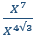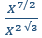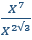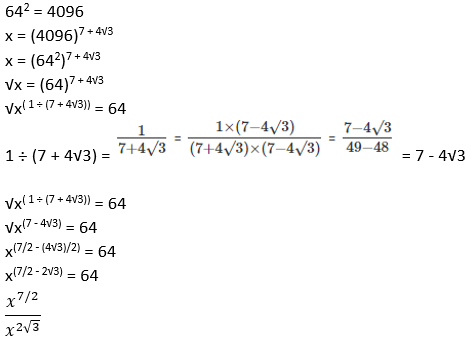# If x = (4096)^7+4√3 | Quantitative Aptitude - CAT Equation

## CAT 2020 - Slot 1 - Quantitative Aptitude - Equation - Question 9 - If x = (4096)^7+4√3

Q. 9: If x = (4096)^7+4√3 , then which of the following equals 64?

##### 2.3.4.### Past Year Question Paper & SolutionsCounselling Session
By IIM Mentor

#### Free Material Area